• Python's curve_fit calculates the best-fit parameters for a function with a single independent variable, but is there a way, using curve_fit or something else, to fit for a function with multiple inde...Python's curve_fit calculates the best-fit parameters for a function with a single independent variable, but is there a way, using curve_fit or something else, to fit for a function with multiple independent variables? For example:
def func(x, y, a, b, c):
return log(a) + b*log(x) + c*log(y)
where x and y are the independent variable and we would like to fit for a, b, and c.
解决方案
You can pass curve_fit a multi-dimensional array for the independent variables, but then your func must accept the same thing. For example, calling this array X and unpacking it to x, y for clarity:
import numpy as np
from scipy.optimize import curve_fit
def func(X, a, b, c):
x,y = X
return np.log(a) + b*np.log(x) + c*np.log(y)
# some artificially noisy data to fit
x = np.linspace(0.1,1.1,101)
y = np.linspace(1.,2., 101)
a, b, c = 10., 4., 6.
z = func((x,y), a, b, c) * 1 + np.random.random(101) / 100
# initial guesses for a,b,c:
p0 = 8., 2., 7.
print curve_fit(func, (x,y), z, p0)
Gives the fit:
(array([ 9.99933937, 3.99710083, 6.00875164]), array([[ 1.75295644e-03, 9.34724308e-05, -2.90150983e-04],
[ 9.34724308e-05, 5.09079478e-06, -1.53939905e-05],
[ -2.90150983e-04, -1.53939905e-05, 4.84935731e-05]]))

展开全文• 比如z=f（x，y），给出（x，y，z）的个数据点，然后怎么拟合出f函数，给出源码就更好了</p>r语言
• 首先夸一下自己上课没有睡着并且...截图为证，什么噪声啊拟合啊我感觉和老师上课讲的内容关联不是很大，或者说只有我听不懂系列， 先讲上课内容吧，这节课主要讲了三个库（算了还是等我先写完作业吧，头好大） ...


python - 作业6：函数拟合
IPythonTab自动完成内省中断正在执行的代码保留命令历史找回忘记的保存的变量魔术命令
numpy库高效调用创建array指定数据类型对数据进行索引和切片操作不复制内容np数组的元素运算数组间运算数组内容审核数组重塑对数组增加内容矩阵运算基本的一些功能random模块matrixmatrix和array的三个主要区别建议
SciPy*10/23更新* 拟合效果*10月24日* 完结更新*2020/02/04*更新code

github指路 冲冲冲冲冲

----------------------------------原文–如下--------------------------------
首先夸一下自己上课没有睡着并且精神面貌极佳，美中不足的是我以为8.55上课所以迟到惹……
从后面开始讲，看到这个作业我都惊呆了，无法理解他的意思= =
截图为证，什么噪声啊拟合啊我感觉和老师上课讲的内容关联不是很大，或者说只有我听不懂系列，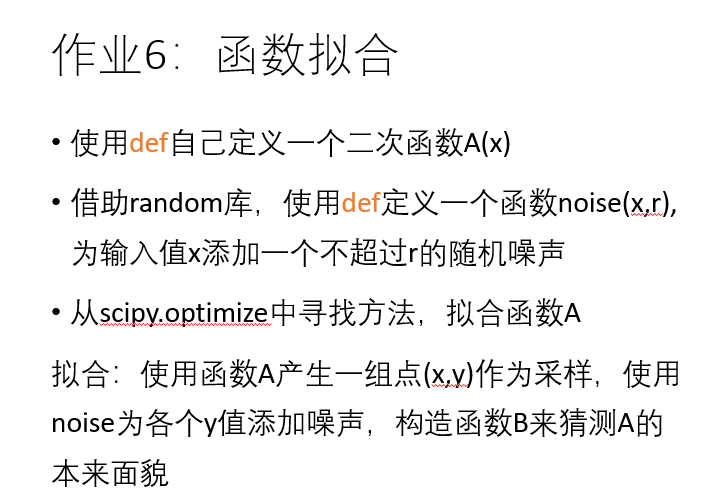先讲上课内容吧，这节课主要讲了三个库（算了还是等我先写完作业吧，头好大） 第一个库 叫
IPython
IPython是一个增强的python shell
这部分我迟到了 ，看了下同桌的笔记 ，提到了它可以让许多python对象的显示形式更友好；有更详细的异常显示；可以增强额外的命令；还有交互式的数据处理
然后对ipython进行了相应的功能介绍，包括：
Tab自动完成 + 内省 + 中断正在执行的代码 + 保留命令历史 + 找回忘记保存的变量 + 超级特殊的魔术命令
一个个来
Tab自动完成
Tab自动完成就是利用Tab键可以得到变量名和函数名或者说是显示模块调用或者你可能用到的常量等等等
内省
内省就是类似于对原始python help的改进，功能差别不大，增加和保留了一点点信息，删掉了类型的一些介绍
中断正在执行的代码
中断正在执行的代码，因为ipython是一个增强的shell ，但他还是一个shell，然后呢，是shell就得进行交互式数据处理，ok这里就会出现一种很想让人避免却很难避免的情况——命令行输错了，运行时间有点长，敲了回车之后不想运行代码了，怎么做呢，在ipython可以按下ctrl+c强制退出上一个命令，造福人类
保留命令历史
保留命令历史，和windows的cmd一样，按上箭头可以得到上一次操作，个人觉得也很实用
第五个
找回忘记的保存的变量
找回忘记的保存的变量。这个是默认的，不管你知道不知道ipython都会帮你做了（上面那个保留命令历史也是）老师给的说明是把最近的两个输出结果保存在名称为‘_’ 和 ‘__’的变量里。但是我有点不懂输出结果的话……计算结果保存与否系列，其实不是很懂，据说在运算时间较长的时候比较适用
魔术命令
高级兮兮的魔术命令，就是以%开头的指令，具体的话👇
上课讲到了一个计算代码运行时间的指令叫%timeit（代码块的话就%%timeit）
运行程序文件 %run（为了写的方便 一点 顺便讲了路径管理功能 也就是%bookmark 还有个改变路径的和cmd很像 %cd）
然后还讲了在交互模式下执行一段代码的命令 %cpaste（这里我没听太全面太明白太理解）……但是呢，老师在课上试了一下 ipython 可以之间复制一段代码在交互模式讲执行= =显然对应的python自带的dell不可以 还有%debug 是用来调试和定位异常的= =
ipython库到此结束
------------------------10/22更新------------------------
numpy库
numpy库通常用来进行高性能计算。前面不知道有没有讲到，python只考虑方便没考虑高效，换言之，两者也很难兼得- -，反正呢python的运行速度较于其他语言就像是在爬（比赛时间其他语言 1000ms，python 2000ms）
高效
提问：为什么numpy库比较高效呢👇
回答：他是建立在C/C++语言的基础上的，所以快呀!
也就是说访问这个库就相当于访问已经编译好的C/C++的库
调用
提问：要怎么调用呢👇
回答：import numpy (as np) (当然这是要在install这个库之后进行的操作)
?然后是numpy里面常见一个数据结构 ： 多维数组 array……
每个array都有两个属性，一个是shape（各维度大小）另一个是dtype（数据类型）
创建array
提问：怎么创建array呢👇
回答：np.array([list]) 这是最基础的方法，当然可以创建一些特殊的矩阵，比如eye方法，可以创建对角线型矩阵，arange方法可以创建一定范围内的一阶矩阵等等等
指定数据类型
提问：如何指定数据类型呢👇
回答：arr1=np.array([1,2,3],dtype=np.float64)
对数据进行索引和切片操作
提问：怎么对数据进行索引和切片操作呢👇
回答：索引和切片操作和原python序列是一样的（2维数组可索引两次），要注意的是索引和切片都部复制内容，需要使用.copy（）方法复制
不复制内容
提问：为什么不复制内容呢👇
回答：前面讲到numpy库是用来进行高性能运算的，所以说尽可能做到能不复制就复制。数据量巨大，会造成复制时间长、占用内存大的不便，也就是说这样不太好
np数组的元素运算
np数组的元素运算 ，就是一元运算 比如abs（）求绝对值，sqrt（）开根号这种类似的方法，
数组间运算
数组间运算，就是双目运算 比如 maxinum（）这种求两个数组里的最大数的方法（好像描述的不是很清楚……改天打个例子试试，理解中的话应该是[1,2,5],[2,3,4] 得到应该是[2,3,5]这个样子？？），
数组内容审核
数组内容审核，就是说对某些数组可能会有元素值缺失这种情况……[1,2,3]比如这样，如果不对其进行判断和处理的话，在程序运行时候就可能会产生一些难以找到的bug，所以就有了这个isnan（）的方法，方法返回一个bool 的数组，找出原数组种那些是NAN的位置.
数组重塑
数组重塑的方法 功能命名 reshape（），拿例子讲话，比如
a1=np.array([1.2.3.4.5.6])
a2=a1.reshape(2,3)
print(a2)

得到a2=[[1,2,3],[4,5,6]]
对数组增加内容
对数组增加内容: np.insert(arr1,p,arr2,axis=0)其中一个参数arr1是指带加入数据的数组，p指得是加入数据的起始位置，arr2是需要加入的数组，axis是指增加的维度值，二维的就是指行和列
矩阵运算
矩阵运算，比如arr.T()就是矩阵转置运算 np.dot(arr1,arr2)就是点乘运算
基本的一些功能
接下来就是基本的一些功能，比如max()找最大值 ，argmax()找最大值的位置
random模块
numpy库里的random模块 ， 比较特殊的是它可以生成符合某种分布的采样，比如说 normal正态分布/ binomial二项分布的采样等等
matrix
接下来！讲了numpy库里的另一个数据结构，也是存储多维数组的，就是matrix嗯。
matrix和array的三个主要区别
①matrix索引返回的是同阶矩阵，array返回的降阶的矩阵 举个栗子： 对于数据都是[[1,2,3],[4,5,6],[7,8,9]]的 a返回的是1 m返回的是[]
②在matrix中，* 运算指的是矩阵乘法
③matrix有个属性I表示它的逆矩阵
然后np.asarray()可以讲matrix转换成array
建议
老师给的一个建议就是能使用numpy库里的操作就不要用python内置的循环和判断语句（原因无他，python在爬）
SciPy
第三个库就是SciPy，据说是用于数学、科学、工程领域的库，可以有效计算Numpy矩阵（这里老师没有过多介绍，可能希望我们能够自学成才吧，老师强调了很多遍学习的技巧，两遍叭？大概？？）
贴张图 表明心意 我希望我能行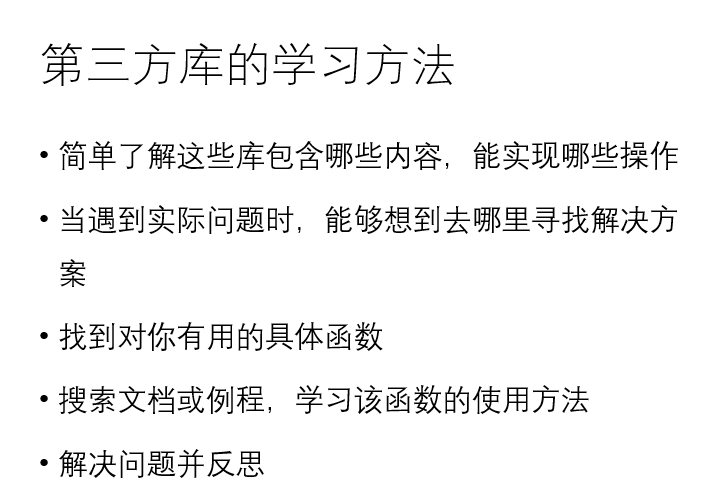10/23更新 拟合效果
然后就是作业了 那个拟合函数 其实我还是不太理解的样子 等我搞明白了就 ……
上课写的代码是利用 scipy库里的optimize模块里的 curve_fit子模块写的一个二次函数，xy的点都是随机的所以效果不是很好， ！！！！然后！！！！
我去截图的时候！！！看起来很不错呀！！！！
这个图，这是我看到的效果（目前为止）最好的一个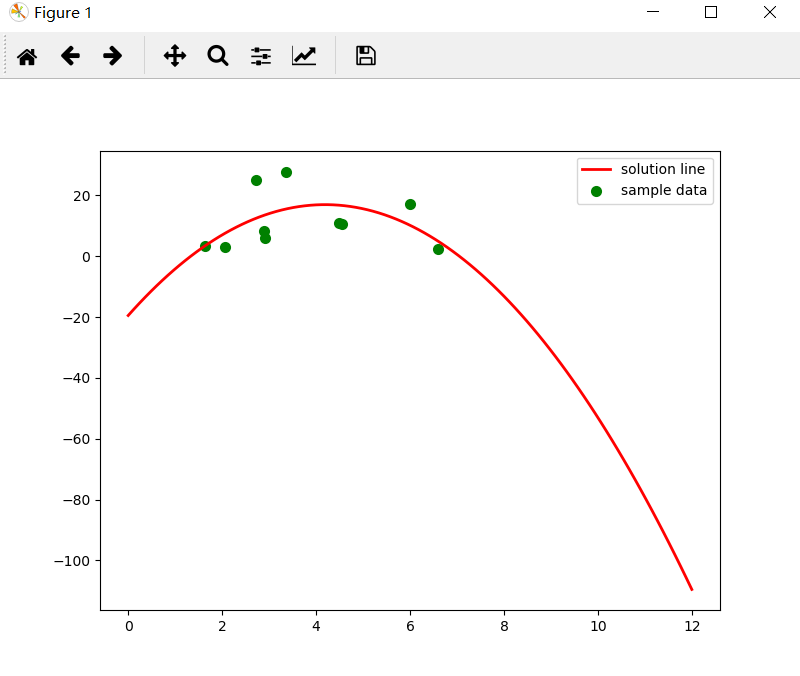这是代码↓
import numpy as np
from scipy.optimize import leastsq
from scipy.optimize import curve_fit
import matplotlib.pyplot as plt
import random
xx = []
yy = []

def A(params, x):
a, b, c = params
return a * x ** 2 + b * x + c

def nosie():
for i in range(0,10):
x = random.uniform(1,10)
y = random.uniform(2,30)
xx.append(x)
yy.append(y)

# ……

def error(params, x, y):
return A(params, x) - y

def slovePara():
p0 = [10, 10, 10]
Para = leastsq(error, p0, args=(X, Y))
return Para

Para = slovePara()
a, b, c = Para

plt.figure(figsize=(8, 6))
plt.scatter(X, Y, color="green", label="sample data", linewidth=2)
x = np.linspace(0, 12, 100)y = a * x * x + b * x + c
plt.plot(x, y, color="red", label="solution line", linewidth=2)
plt.legend()
plt.show()

然后，呢，我就想做一个效果好一点的拟合曲线
于是在w同学的帮助下
我得到了这样的效果图，用指数函数来拟合= =，利用 scipy库里的optimize模块里的 curve_fit子模块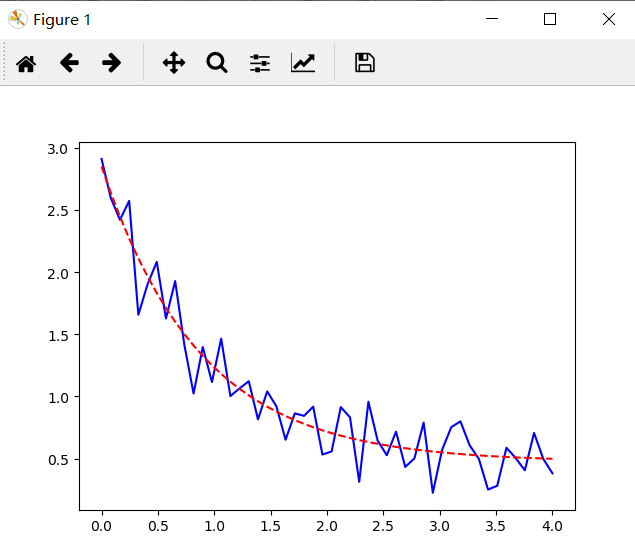10月24日 完结更新
我对其进行了改进所以现在的代码大概长成这个样子：
#!/usr/bin/env python
# -*- coding: utf-8 -*-
# @Time : 2019/10/22 10:37
# @Author : Chen Shan

import numpy as np
from scipy.optimize import leastsq
from scipy.optimize import curve_fit
import matplotlib.pyplot as plt
import random

# 1 ----------二次函数拟合，point （x，y）随机生成
xx = []
yy = []
def A(params, x):
a, b, c = params
return a * x ** 2 + b * x + c

def nosie():
for i in range(0,10):
x = random.uniform(1,10)
y = random.uniform(2,30)
xx.append(x)
yy.append(y)

# ……
def error(params, x, y):
return A(params, x) - y

def slovePara():
p0 = [10, 10, 10]
Para = leastsq(error, p0, args=(X, Y))
return Para

Para = slovePara()
a, b, c = Para
# print("a = "+ str(a) + ", b = "+ str(b) + ", c = "+ str(c))
# print("cost:" + str(Para))
print("y = " + str(round(a, 2)) + "x*x+" + str(round(b, 2)) + "x+" + str(c))

plt.figure(figsize=(8, 6))
plt.scatter(X, Y, color="green", label="sample data", linewidth=2)

x = np.linspace(0, 12, 100)
y = a * x * x + b * x + c
plt.plot(x, y, color="red", label="solution line", linewidth=2)
plt.legend()
plt.show()

# # 2----------指数函数拟合，point x 随机生成
# def A2(x, a, b, c):
#     return a * np.exp(-b * x) + c
# # nosie
# xdata = np.linspace(0, 4, 50)
# y = A2(xdata, 2.5, 1.3, 0.5)
# ydata = y + 0.11 * np.random.normal(size=len(xdata))
#
# plt.plot(xdata, ydata, 'b-')
#
# popt, pcov = curve_fit(A2, xdata, ydata)
#
# y2 = [A2(i, popt, popt, popt) for i in xdata]
# plt.plot(xdata, y2, 'r--')
#
# print("y = "+str(popt)+" * np.exp(-"+str(popt)+" * x) + "+str(popt))
#
# plt.show()


2020/02/04更新code
#!/usr/bin/env python
# -*- coding: utf-8 -*-
# @Time : 2019/10/22 10:37
# @Author : Chen Shan
# Function : Randomly generate 50 points, fit binary function and present

import numpy as np
from scipy.optimize import leastsq
from scipy.optimize import curve_fit
import matplotlib.pyplot as plt
import random

# 1 ----------二次函数拟合，point （x，y）随机生成
xx = []
yy = []
def A(params, x):
a, b, c = params
return a * x ** 2 + b * x + c

def nosie():
for i in range(0,10):
x = random.uniform(1,10)
y = random.uniform(2,30)
xx.append(x)
yy.append(y)

nosie()
X = np.array(xx)
Y = np.array(yy)

# 误差函数，即拟合曲线所求的值与实际值的差
def error(params, x, y):
return A(params, x) - y

# 对参数求解
def slovePara():
p0 = [10, 10, 10]
Para = leastsq(error, p0, args=(X, Y))
return Para

Para = slovePara()
a, b, c = Para
# print("a = "+ str(a) + ", b = "+ str(b) + ", c = "+ str(c))
# print("cost:" + str(Para))
print("y = " + str(round(a, 2)) + "x*x+" + str(round(b, 2)) + "x+" + str(c))

plt.figure(figsize=(8, 6))
plt.scatter(X, Y, color="green", label="sample data", linewidth=2)

x = np.linspace(0, 12, 100)
y = a * x * x + b * x + c
plt.plot(x, y, color="red", label="solution line", linewidth=2)
plt.legend()
plt.show()

# # 2----------指数函数拟合，point x 随机生成
# def A2(x, a, b, c):
#     return a * np.exp(-b * x) + c
# # nosie
# xdata = np.linspace(0, 4, 50)
# y = A2(xdata, 2.5, 1.3, 0.5)
# ydata = y + 0.11 * np.random.normal(size=len(xdata))
#
# plt.plot(xdata, ydata, 'b-')
#
# popt, pcov = curve_fit(A2, xdata, ydata)
# # popt数组中，三个值分别是待求参数a,b,c
# y2 = [A2(i, popt, popt, popt) for i in xdata]
# plt.plot(xdata, y2, 'r--')
#
# print("y = "+str(popt)+" * np.exp(-"+str(popt)+" * x) + "+str(popt))
#
# plt.show()


展开全文scipy optimize
• (二)、获得拟合函数并绘制图象 (三)、通过计算拟合优度评价拟合函数 (四)、综合代码 (五)、结果输出 关于Python数据分析在数学建模中的更相关应用： Python数据分析在数学建模中的应用汇总（持续更新中！） ...


文章目录
(一)、读取数据(二)、获得拟合函数并绘制图象(三)、通过计算拟合优度评价拟合函数(四)、综合代码(五)、结果输出

关于Python数据分析在数学建模中的更多相关应用：Python数据分析在数学建模中的应用汇总（持续更新中！）

(一)、读取数据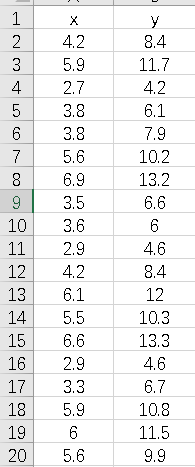#从excel文件中读取数据
wb = xlrd.open_workbook(filename=file)#打开文件
sheet = wb.sheet_by_index(0)#通过索引获取表格
rows = sheet.nrows # 获取行数
all_content = []        #存放读取的数据
for j in range(0, 2):       #取第1~第2列对的数据
temp = []
for i in range(1,rows) :
cell = sheet.cell_value(i, j)   #获取数据
temp.append(cell)
all_content.append(temp)    #按列添加到结果集中
temp = []
return np.array(all_content)    #返回读取的数据

(二)、获得拟合函数并绘制图象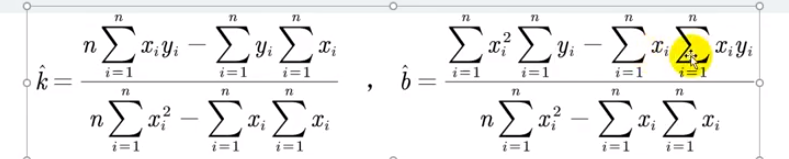#获得拟合函数并绘制图象
def temp1(datas):
x = datas        #获取自变量x
y = datas        #获取因变量y
n = np.size(answer1, axis = 1)  #获取有多少个自变量，axis=1代表获取矩阵的列数
#根据公式计算k
k = (n*np.sum(x*y) - np.sum(x)*np.sum(y)) / (n*np.sum(np.power(x,2)) - np.sum(x) * np.sum(x))
#根据公式计算b
b = (np.sum(np.power(x,2)) * np.sum(y) -np.sum(x) * np.sum(x*y)) / (n*np.sum(np.power(x,2)) - np.sum(x) * np.sum(x))
las = k*x + b       #根据公式得到拟合函数
fig = plt.figure()  #获得figure对象
ax1.set_xlim([min(x)-0.5, max(x)+0.5])      #设置x轴刻度
ax1.set_ylim([min(y) -0.5, max(y) +0.5])    #设置y轴刻度
plt.plot(x,las,'k',label='拟合函数')    #画出拟合函数
plt.plot(x,y,'o',label = '样本数据')    #画出样本数据
plt.grid()  #添加网格线
ax1.legend(loc = 'best')    #设置图例的位置为最佳best
return [k,b]    #返回k和b

(三)、通过计算拟合优度评价拟合函数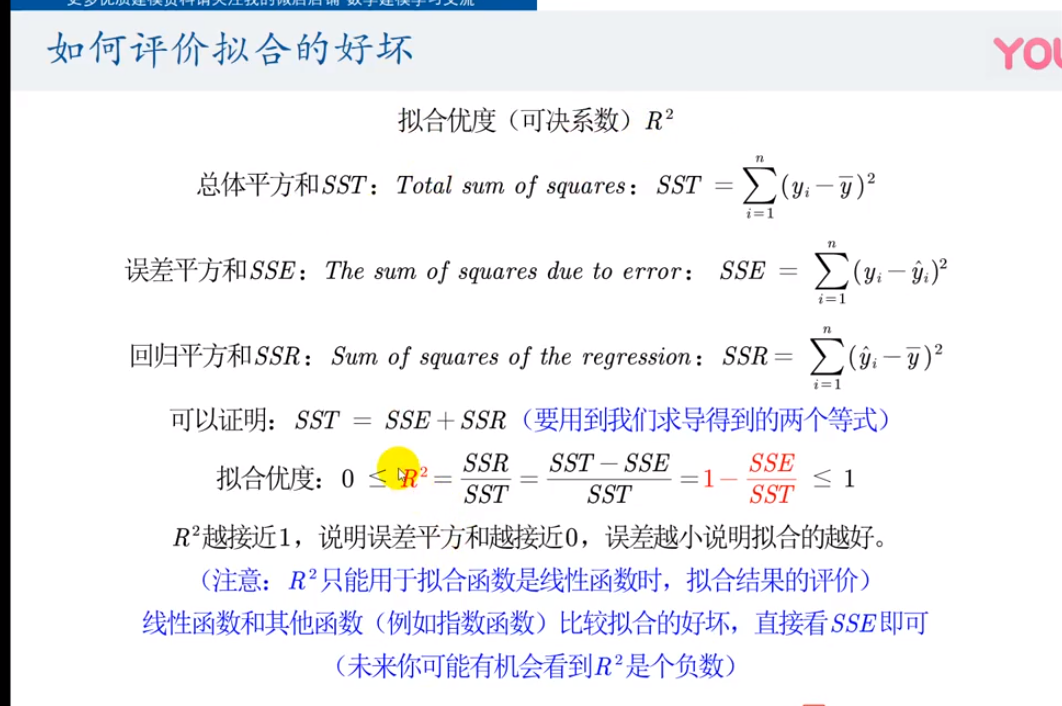#通过计算拟合优度评价拟合函数
def judge(datas,k,b):
x = datas    #获取自变量x
y = datas    #获取自变量y
z = k * x + b   #得到拟合函数
SST = np.sum(np.power(y - np.average(y),2))     #根据公式计算SST
SSE = np.sum(np.power(y - z, 2))                #根据公式计算SSE
SSR = np.sum(np.power(z - np.average(y),2))     #根据公式计算SSR
R_2 = SSR / SST             #得到R_2
print('k = ',k)
print('b = ',b)
print('SST = ',SST)
print('SSE = ',SSE)
print('SSR = ',SSR)
print('R_2 = ',R_2)

(四)、综合代码
# -*- coding: utf-8 -*-
"""
Created on Mon Jul 29 11:03:49 2019

@author: lenovo
"""
import xlrd
import numpy as np
import matplotlib.pyplot as plt

#从excel文件中读取数据
wb = xlrd.open_workbook(filename=file)#打开文件
sheet = wb.sheet_by_index(0)#通过索引获取表格
rows = sheet.nrows # 获取行数
all_content = []        #存放读取的数据
for j in range(0, 2):       #取第1~第2列对的数据
temp = []
for i in range(1,rows) :
cell = sheet.cell_value(i, j)   #获取数据
temp.append(cell)
all_content.append(temp)    #按列添加到结果集中
temp = []
return np.array(all_content)    #返回读取的数据
#获得拟合函数并绘制图象
def temp1(datas):
x = datas        #获取自变量x
y = datas        #获取因变量y
n = np.size(answer1, axis = 1)  #获取有多少个自变量，axis=1代表获取矩阵的列数
#根据公式计算k
k = (n*np.sum(x*y) - np.sum(x)*np.sum(y)) / (n*np.sum(np.power(x,2)) - np.sum(x) * np.sum(x))
#根据公式计算b
b = (np.sum(np.power(x,2)) * np.sum(y) -np.sum(x) * np.sum(x*y)) / (n*np.sum(np.power(x,2)) - np.sum(x) * np.sum(x))
las = k*x + b       #根据公式得到拟合函数
fig = plt.figure()  #获得figure对象
ax1.set_xlim([min(x)-0.5, max(x)+0.5])      #设置x轴刻度
ax1.set_ylim([min(y) -0.5, max(y) +0.5])    #设置y轴刻度
plt.plot(x,las,'k',label='拟合函数')    #画出拟合函数
plt.plot(x,y,'o',label = '样本数据')    #画出样本数据
plt.grid()  #添加网格线
ax1.legend(loc = 'best')    #设置图例的位置为最佳best
return [k,b]    #返回k和b
#通过计算拟合优度评价拟合函数
def judge(datas,k,b):
x = datas    #获取自变量x
y = datas    #获取自变量y
z = k * x + b   #得到拟合函数
SST = np.sum(np.power(y - np.average(y),2))     #根据公式计算SST
SSE = np.sum(np.power(y - z, 2))                #根据公式计算SSE
SSR = np.sum(np.power(z - np.average(y),2))     #根据公式计算SSR
R_2 = SSR / SST             #得到R_2
print('k = ',k)
print('b = ',b)
print('SST = ',SST)
print('SSE = ',SSE)
print('SSR = ',SSR)
print('R_2 = ',R_2)


(五)、结果输出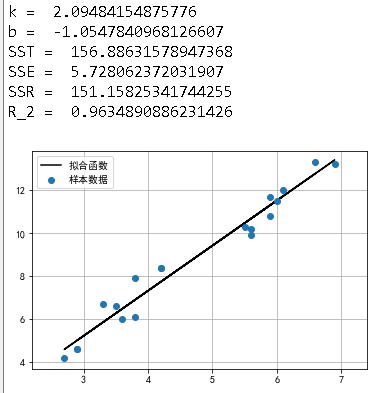展开全文数学建模
• from mpl_toolkits.mplot3d import Axes3D # 3D 绘图 def func(x, y, p): """ 数据拟合所用的函数：z=ax+by :param x: 自变量 x :param y: 自变量 y :param p: 拟合参数 a, b """ a, b, c = p return a * x**...
1、一元多项式
功能：最小二乘法拟合一元线性多项式 p(1)*x**n+p(2)*x**(n-1)*...*p(n-1)*x+p(n)，指数不能是小数   模块：numpy   代码如下：
import matplotlib.pyplot as plt
import numpy as np

x = np.arange(1, 17, 1)
y = np.array([4.00, 6.40, 8.00, 8.80, 9.22, 9.50, 9.70, 9.86, 10.00, 10.20, 10.32, 10.42, 10.50, 10.55, 10.58, 10.60])
z1 = np.polyfit(x, y, 3)#用3次多项式拟合，输出系数从高到0
p1 = np.poly1d(z1)#使用次数合成多项式
yvals=p1(x)

plt.plot(x,y,'*')
plt.plot(x,yvals)
plt.show()
计算结果：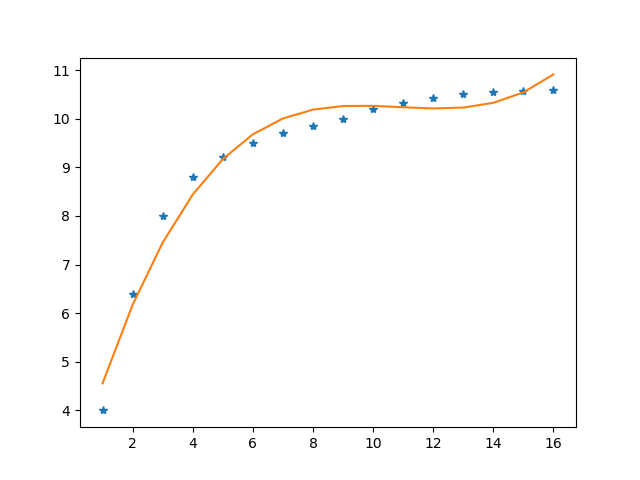#np.poly1d函数解释     1）p1 = np.poly1d([1,2,3])使用系数合成多项式 1*x**2+2*x**1+3*x**0     2）p1 = np.poly1d([1,2,3],True)使用根合成多项式(x-1)*(x-2)*(x-3)     3）p1(0.5)表示当x = 0.5时，多项式的值为多少     4）p1.r表示当多项式为 0 时，此等式的根     5）p1.c表示生成多项式的系数数组     6）p1.order表示返回最高项的次方数     7）p1表示返回第一项的系数     8）多项式支持实数的四则运算
2、一元任意函数
功能：最小二乘法拟合一元任意函数 y=f(x)   模块：from scipy import optimize   代码如下：
import numpy as np
import matplotlib.pyplot as plt
from scipy import optimize

def func(x,a,b):#需要拟合的函数
return a*np.exp(b/x)

# 拟合点
x0 = [1, 2, 3, 4, 5]
y0 = [1, 3, 8, 18, 36]

a4, b4= optimize.curve_fit(func, x0, y0)
x4 = np.arange(1, 6, 0.01)
y4 = a4*np.exp(b4/x4)

plt.figure()
plt.scatter(x0[:], y0[:], 25, "red")
plt.plot(x4, y4, "purple")
plt.show()
计算结果：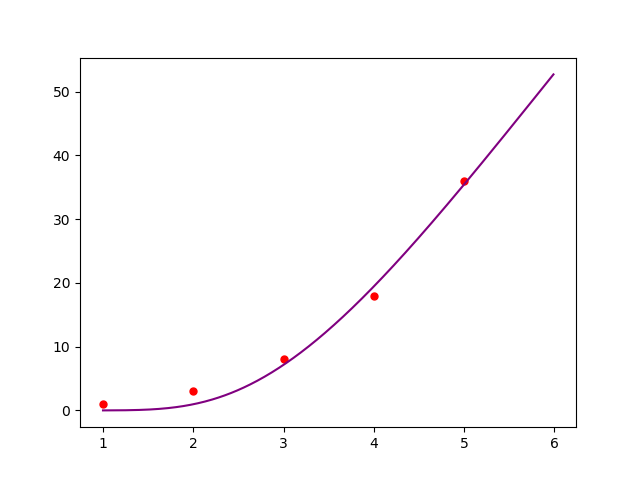存在问题：bounds=[[],[]]，指定参数范围，长度是1或者和参数一样多，为1时表示所有参数都是这个数，[0,[20,30]]即0<a<20,0<b<30     不能返回残差，如何指定迭代结果？
2、任意函数
功能：最小二乘法任意函数   模块：from scipy import optimize   代码如下：
import numpy as np
from scipy import optimize		# 最小二乘法拟合
import matplotlib.pyplot as plt 	# python matplotlib 绘图
from mpl_toolkits.mplot3d import Axes3D  # 3D 绘图

def func(x, y, p):
""" 数据拟合所用的函数：z=ax+by
:param x: 自变量 x
:param y: 自变量 y
:param p: 拟合参数 a, b
"""
a, b, c = p
return a * x**2 + b * y +c*x

def residuals(p, z, x, y):
""" 得到数据 z 和拟合函数之间的差
"""
print(p)
return z - func(x, y, p)

def main():
x = np.arange(5)
y = np.arange(5)
z = np.array([2, 4, 7, 7, 10])  # 数据随便取的

plsq = optimize.leastsq(residuals, np.array([0, 0, 0]), args=(z, x, y))  # 最小二乘法拟合
# [0, 0] 为参数 a, b 初始值

a, b, c= plsq  # 获得拟合结果
print("拟合结果:\na = {}".format(a))
print("b = {}".format(b))
print("c = {}".format(c))

# 绘图
xp = np.linspace(-1, 6, 100)
yp = np.linspace(-1, 6, 100)

X, Y = np.meshgrid(xp, yp)
Z = func(X, Y, [a, b, c])   # 带入拟合得到的 a, b, c

fig = plt.figure(figsize=(8, 6))
ax = Axes3D(fig)    # 3D 绘图

ax.plot_surface(X, Y, Z, alpha=0.5)
ax.scatter(x, y, z, color="r")

ax.set_xlabel("x")
ax.set_ylabel("y")
ax.set_zlabel("z")

plt.show()

main()

展开全文• python实现描述性统计、频数分布图、正态分布检验、概率密度曲线拟合 from scipy.stats import chi2 # 卡方分布 from scipy.stats import norm # 正态分布 from scipy.stats import t # t分布 from scip...数据分析 正态分布检验
• 数据分析中经常会使用到数据拟合，本文中将阐述如何实现一元以及多元的线性拟合以及多项式拟合，本文中只涉及实现方式，不涉及理论知识。 模型拟合中涉及的误差评估方法如下所示： import numpy as np def stdError...数据分析
• 如图，x轴是时间，y,z轴表示变量，随着时间是上升——平稳——下降的趋势，怎么求出y和z之间的分段函数有问必答
• 利用给定的自变量和因变量，按指定的最高次方拟合多项式 利用到的知识numpy库的一些函数 ①numpy.arange(1,21) 返回一个1-20的数组 ②numpy.random.rand(20) 返回一个0-1长度为20的数组 ③numpy.ployfit(x,y1,1) ...
• 最近，使用curve_fit时遇到一个问题，百思不得其解，看了官网，上网查都没有找到这种问题所在，最后通过一些实验确定：应该...在做交通流三参数模型拟合时，我使用了scipy的curve_fit函数。数据大概是这个样子的： ...curve_fit
• 广告关闭腾讯云11.11云上盛惠 ，精选热门产品助力上云，云服务器首年88元起，买的越返的越，最高返5000元！下面是我在文本文件中绘制数据散点图的代码。 我打开的文件包含两列。 左列为x坐标，右列为y坐标。 ...
• 所以打算写一个含有十种函数的拟合模型，去看那种拟合的r2最高，将r2最高的函数函数作为最终拟合函数。 一、最后的成果 用n 组数据生产 n组数据 拟合十种回归，保留r2最大的画出期曲线和散点图，标注好函数公式...机器学习
• 小程序储备：散点拟合 #! /usr/bin/env python # -*- coding: utf-8 -*- """ This program is debugged by Harden Qiu """ import numpy as np import matplotlib.pyplot as plt x = np.array([-4,-3,-2,-1,0...
• 曲线拟合：字面意思 应用：预测和检验 方法：两种 最小二乘法（数值不精确） 插值法（数值精确，曲线过每个点） 基础数学理论： 泰勒级数（预测下一个函数值），有限差商，均值，标注差（方差开根），残差平方和...
• 如 电视机和收音机价格销售额的影响，此时自变量有两个。 python 解法： import numpy as np import pandas as pd #import statsmodels.api as sm #方法一 import statsmodels.formula.api as smf #方法二 import...
• 数据拟合的最小二乘法 《计算机科学计算》张宏伟 第二版 第五章习题13 用最小二乘法求拟合下列数据的二次多项式 xi yi 1 10 3 5 4 4 5 2 6 1 7 1 8 2 9 3 10 4 import numpy as np # 默认...
• 3.34 1.5\leq Q_{i} \leq 3.34 1.5≤Qi​≤3.34    W i = 0 或 1 W_{i} = 0或1 Wi​=0或1   实现遗传算法主要有这样几步，第一步，编码 编码   为了满足精度的同时避免使用小数点，我们需要将自变量 Q i Q_{i}...遗传算法
• 目录在下自我介绍线性回归问题的简述拟合是什么？新的改变功能快捷键合理的创建标题，有助于目录的生成如何改变文本的样式插入链接与图片如何插入一段漂亮的代码片生成一个适合你的列表创建一个表格设定内容居中、...机器学习 线性回归
• 梯度下降法 梯度下降法的基本思想可以类比为一个下山的过程。 假设这样一个场景：一个人被困在山上，需要从山上下来(找到山的最低点，也就是山谷)。但此时山上的浓雾很大，导致...首先，我们有一个可微分的函数。这个
• ## python数据拟合fit

千次阅读 2018-12-18 10:52:49
第一部分：多项式拟合 1）一次式 import numpy as np import matplotlib.pyplot as plt x,y = [1,1.25,1.33,1.60,2.0,2.4,2.5,3.0,3.2],[931.525,1163.97,1238.35,1489.44,1861.48\ ,2233.6,2326.64,...
• 深度学习框架：Tensorflow 0.8.0 Python：2.7.6关于LSTM的基础理解参考牛津大学的机器学习视频：Lecture 11 RNN&&LSTM, ... ①data和label是同一个变量，整个模型相当于回归（本文先演示第一种）深度学习 LSTM tensorflow
• 一元线性回归拟合 例子如下: #!/usr/bin/env python # -*- coding:utf-8 -*- # Author's_name_is_NIKOLA_SS # 导入sklearn库下的linear_model类 ...# 转化自变量数据为矩阵 x = [[0.8],, , , [8.6]]机器学习 逻辑回归 人工智能 深度学习
• ## python曲线拟合

千次阅读 2019-08-23 19:29:13
python中曲线拟合： 一个是numpy中的polyfit()函数，多项式拟合，给定变量x、y、多项式次数，...给定变量x、y、拟合函数，返回值有两个，popt是拟合函数中的一维参数array, pcov为拟合函数中参数的 协方差array ; ...
• 多变量的线性回归？好像发现了什么？重新理清一下思路。什么是多项式拟合？对的，这个问题以前没有好好思考过，以前的直观感觉是，不就是给一些x，y的点，让一个多项式去拟合，使得这个多项式的曲线看起来大致符合...
• 从简单的二维线性拟合入手。本文只解决一个问题：在二维平面中找到一条最合适的线，来拟合所有给出的点。...最小二乘法的目的是找到因变量与自变量 之间的函数关系 。对于本文讨论的为题，可以将点的横坐标看做自...
• ## 使用Python估计数据概率分布函数

万次阅读 多人点赞 2016-11-23 16:10:47
不过也是，别人明明是一个类指数函数，你非要哪个二次函数拟合=。= Plan B 在查阅一番以后，发现，对于这类函数拟合的话，from scipy.optimize import curve_fit这个对于高斯型、指数型函数拟合效果...概率分布 数据
• 拟合函数有两个系数，而只有一个点可用于拟合时，虽然在求解最优系数时，仍有两个偏导数方程，但这两个方程线性相关，其实只有一个方程是有效的，因为只有一个点进行拟合，所以这时系数a、b无解或有无穷解。...多项式 方程组求解 梯度下降
• 本文实例讲述了Python实现曲线拟合操作。分享给大家供大家参考，具体如下： 这两天学习了用python拟合曲线。 一、环境配置 本人比较比较懒，所以...且其目录为C:\Python27\ArcGIS10.2，所以引用环境变量时，要注意。
• 文章目录线性相关皮尔逊相关系数（stats.pearsonr）斯皮尔曼相关系数（stats.spearmanr）线性拟合/回归最小二乘法（optimize.least_squares）R方(R squared)代码实现 线性相关 线性相关分析是描述两变量间直线相关，......

# python多自变量拟合函数python 订阅